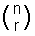## Title

Binomial Distribution
Quick revise

If a discrete random variable X has the following probability density function (p.d.f.), it is said to have a binomial distribution:

• P(X = x) = nCx q(n-x)px, where q = 1 - p

p can be considered as the probability of a success, and q the probability of a failure.

Note: nCr (“n choose r”) is more commonly written, but I shall use the former because it is easier to write on a computer. It means the number of ways of choosing r objects from a collection of n objects (see permutations and combinations).

If a random variable X has a binomial distribution, we write X ~ B(n, p) (~ means ‘has distribution…’).

n and p are known as the parameters of the distribution (n can be any integer greater than 0 and p can be any number between 0 and 1). All random variables with a binomial distribution have the above p.d.f., but may have different parameters (different values for n and p).

Example

A coin is thrown 10 times. Find the probability density function for X, where X is the random variable representing the number of heads obtained.

The probability of throwing a head is ½ and the probability of throwing a tail is ½. Therefore, the probability of throwing 8 tails is (½)8

If we throw 2 heads and 8 tails, we could have thrown them HTTTTTHTT, or TTHTHTTTTT, or in a number of other ways. In fact, the total number of ways of throwing 2 heads and 8 tails is 10C2 (see the permutations and combinations section).

Hence the probability of throwing 2 heads and 8 tails is 10C2× (½)2× (½)8 . As you can see this has a Binomial distribution, where n = 10, p = ½.

You can see, therefore, that the p.d.f. is going to be: P(X = x) = 10Cx (½)(10-x) (½)x .

From this, we can work out the probability of throwing, for example, 3 heads (put x = 3).

Expectation and Variance

If X ~ B(n,p), then the expectation and variance is given by:

• E(X) = np

• Var(X) = npq

Example

In the above example, what is the expected number of heads thrown?

E(X) = np

Now in the above example, p = probability of throwing a head = ½ . n = number of throws = 10

Hence expected number of heads = 5.

This is what you would expect: if you throw a coin 10 times you would expect 5 heads and 5 tails on average.

Rate: